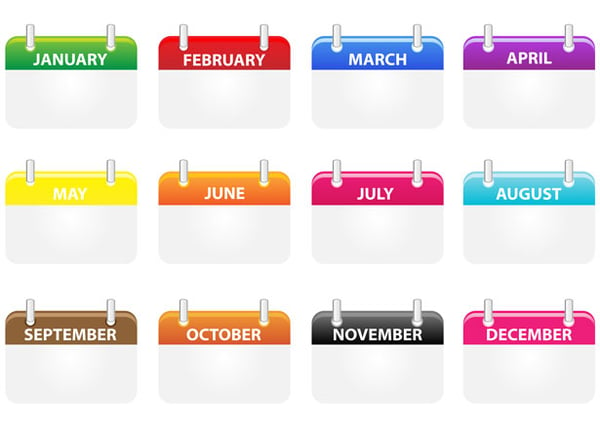Related Tags

calendar
date
time
java
abstract class

# Learning about the Calendar class in JavaEducative Answers Team

Have you ever found yourself sitting and calculating how many more months to a holiday? Or wondering what the day was on the date that you were born, years ago?

The calendar class in Java is an abstract class that allows you to manipulate date and time with various calendar methods and instances.

As this class is an abstract class, you must get instances of the time and date as opposed to calling them using constructors.## What fields does this class have?

The Calendar class has multiple attributes that already exist within it. A few of them are listed below:

• DATE: This is a static, int value that represents the day in a month.

• DAY: This is a static, int value that represents the day in a month. This is also shown by DAY_OF_MONTH. If you want to see the day in the week, the field is called DAY_OF_WEEK and for year, it is DAY_OF_YEAR.

• JANUARY to DECEMBER: Each month name in capital letters represents the number of the month in the year.

• YEAR: This is the static, int value of the year.

• AM_PM: This indicates the time frame for a given time.

• MONDAY to SUNDAY: The name of the weekday in capital letters shows the number the day holds in a week.

Now that we know some of the fields present in this class. Let’s look at a few of the methods and how they can be used.

## Using methods from the calendar class

### 1. Getting an instance of the class

This method is used to get an instance of the date-time, which can then be further manipulated. We have simply printed the time from the entire calendar to make the output simple.

Note: The java.util.* library must be imported for the calendar class to work.

import java.util.*;

class HelloWorld {
public static void main( String args[] ) {
Calendar calendar_demo = Calendar.getInstance();
System.out.println(calendar_demo.getTime());
}
}

Note: The getTime() method simply returns the time object of the calendar_demo.

### 2. Adding to calendar fields

Now that we know how to get an instance of a class, we can use that to manipulate time by adding values to it. Let’s see how we can access the YEAR and TIME fields in the class and manipulate them.

For each example, whichever property is called within the add method, that property is modified by adding the value of the second argument to it. Hence, on line 7 10 years are added to the current date. On line 11, the now modified date has 5 days subtracted from it.

import java.util.*;

class HelloWorld {
public static void main( String args[] ) {
Calendar calendar_demo = Calendar.getInstance();
// Adding 10 years to the date
System.out.println(calendar_demo.getTime());

// Subtracting 5 days from the date
System.out.println(calendar_demo.getTime());
}
}

### 3. Finding the maximum and minimum values

The Calendar class also allows the user to find the maximum and minimum possible values that a field in the class can have. The code snippets below show how you can find these.

For each example, the maximum and minimum are found for the attribute given to the relevant method. Hence, on line 7, the method returns the highest number of days possible in a year. On line 11, the method returns the least number of days a month must have.

import java.util.*;

class HelloWorld {
public static void main( String args[] ) {
Calendar calendar_demo = Calendar.getInstance();
// The maximum possible number of days in a year
int maximum = calendar_demo.getMaximum(calendar_demo.DAY_OF_YEAR);
System.out.println("The maximum number of days in a year: " + maximum);

// The minimum possible number of days in a month
int minimum = calendar_demo.getMinimum(calendar_demo.DAY_OF_MONTH);
System.out.println("The minimum number of days in a month: " + minimum);
}
}

RELATED TAGS

calendar
date
time
java
abstract class